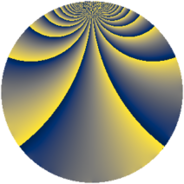# Properties

 Label 1815.2.mLevel $1815$ Weight $2$ Character orbit 1815.m Rep. character $\chi_{1815}(511,\cdot)$ Character field $\Q(\zeta_{5})$ Dimension $288$ Sturm bound $528$

# Related objects

## Defining parameters

 Level: $$N$$ $$=$$ $$1815 = 3 \cdot 5 \cdot 11^{2}$$ Weight: $$k$$ $$=$$ $$2$$ Character orbit: $$[\chi]$$ $$=$$ 1815.m (of order $$5$$ and degree $$4$$) Character conductor: $$\operatorname{cond}(\chi)$$ $$=$$ $$11$$ Character field: $$\Q(\zeta_{5})$$ Sturm bound: $$528$$

## Dimensions

The following table gives the dimensions of various subspaces of $$M_{2}(1815, [\chi])$$.

Total New Old
Modular forms 1152 288 864
Cusp forms 960 288 672
Eisenstein series 192 0 192

## Trace form

 $$288q - 8q^{2} - 80q^{4} - 8q^{7} + 16q^{8} - 72q^{9} + O(q^{10})$$ $$288q - 8q^{2} - 80q^{4} - 8q^{7} + 16q^{8} - 72q^{9} + 16q^{10} + 4q^{13} - 8q^{14} - 72q^{16} + 16q^{17} + 12q^{18} - 8q^{19} - 16q^{20} + 40q^{23} + 36q^{24} - 72q^{25} - 40q^{26} - 28q^{28} - 8q^{29} - 4q^{30} - 20q^{31} - 56q^{32} + 56q^{34} - 8q^{35} - 80q^{36} + 44q^{37} + 28q^{38} - 8q^{39} - 12q^{40} + 28q^{41} - 40q^{42} + 40q^{43} - 24q^{46} + 16q^{47} - 32q^{48} - 92q^{49} - 8q^{50} + 4q^{51} - 52q^{52} - 24q^{53} + 96q^{56} - 8q^{57} + 4q^{58} - 88q^{59} - 20q^{61} - 16q^{62} - 8q^{63} - 68q^{64} - 24q^{65} + 40q^{67} + 40q^{68} - 24q^{69} + 20q^{70} - 48q^{71} - 4q^{72} + 36q^{73} + 84q^{74} + 40q^{76} - 64q^{78} + 32q^{79} - 32q^{80} - 72q^{81} + 28q^{82} + 4q^{83} + 48q^{84} - 8q^{85} + 40q^{86} + 88q^{87} + 160q^{89} - 4q^{90} + 68q^{91} + 56q^{92} + 40q^{93} + 36q^{94} + 12q^{96} - 20q^{97} - 96q^{98} + O(q^{100})$$

## Decomposition of $$S_{2}^{\mathrm{new}}(1815, [\chi])$$ into newform subspaces

The newforms in this space have not yet been added to the LMFDB.

## Decomposition of $$S_{2}^{\mathrm{old}}(1815, [\chi])$$ into lower level spaces

$$S_{2}^{\mathrm{old}}(1815, [\chi]) \cong$$ $$S_{2}^{\mathrm{new}}(33, [\chi])$$$$^{\oplus 4}$$$$\oplus$$$$S_{2}^{\mathrm{new}}(55, [\chi])$$$$^{\oplus 4}$$$$\oplus$$$$S_{2}^{\mathrm{new}}(121, [\chi])$$$$^{\oplus 4}$$$$\oplus$$$$S_{2}^{\mathrm{new}}(165, [\chi])$$$$^{\oplus 2}$$$$\oplus$$$$S_{2}^{\mathrm{new}}(363, [\chi])$$$$^{\oplus 2}$$$$\oplus$$$$S_{2}^{\mathrm{new}}(605, [\chi])$$$$^{\oplus 2}$$